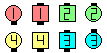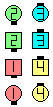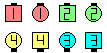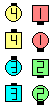Definitions of Square Dance Calls and Concepts
Gee Whiz [C3B]Index -->  Plus  |  A1  |  A2  |  C1  |  C2  |  C3A  |  C3B  |  C4  |  NOL  |
Definitions (Text Only) -->  Plus  |  A1  |  A2  |  C1  |  C2  |  C3A  |  C3B  |  C4  |  NOL  |
 Find call:

 \$B8@8l(BFrom Parallel Lines. EN: 10
\$BJ?9T\$J(B Line \$B\$+\$i(B. JP: 10

Out-Facing Couple (or the designated dancers) do your part Cross Fire as the Others do your part Vertical Tag The LineEN: 20
\$B30\$r8~\$\$\$?%+%C%W%k(B (\$B\$^\$?\$O;X<(\$5\$l\$??M(B) \$B\$O(B Cross Fire \$B\$N<+J,\$N%Q!<%H\$r9T\$\$(B, \$BB>\$N?M\$O(B Vertical Tag The Line \$B\$r9T\$\$\$^\$9(B. JP: 20

Ends in Columns. EN: 30
Column \$B\$G=*\$o\$j\$^\$9(B. JP: 30Gee Whiz\$B\$NA0(B \$B8e(B

\$BCm

• The original definition of Gee Whiz describes the lead Couple's part as a 1/2 Wheel Across, Step Thru, and Face In. Our definition uses a Cross Fire as a concise way to state this movement. EN: 40
Gee Whiz \$B\$N%*%j%8%J%k\$NDj5A\$G\$O(B lead Couple \$B\$NF0\$-\$r(B 1/2 Wheel Across, Step Thru, Face In \$B\$H5-=R\$7\$F\$\$\$^\$9(B. \$B;dC#\$NDj5A\$G\$O(B Cross Fire \$B\$r;H\$C\$F4J7i\$K\$7\$F\$\$\$^\$9(B. JP: 40
• From Out-Facing Lines, the caller may designate which Couple is to do the Cross Fire (e.g., 'Right Gee Whiz' means the R-H Couple Cross Fire as the L-H Couple Vertical Tag The Line). If the caller says 'No one Gee Whiz', then everyone does the Vertical Tag The Line. If the caller does not designate anyone or says 'Everyone Gee Whiz', then everyone does the Cross Fire (ending in a Double Pass Thru formation). Note that Right Gee Whiz ends in L-H Columns, whereas Left Gee Whiz ends in R-H ColumnsEN: 50
\$B30\$r8~\$\$\$?(B Line \$B\$+\$i(B, \$B\$I\$A\$i\$N%+%C%W%k\$,(B Cross Fire \$B\$r\$9\$k\$+\$O%3!<%i!<\$,;XL>\$9\$k\$G\$7\$g\$&(B (\$BNc\$(\$P(B, 'Right Gee Whiz' \$B\$O(B \$B1&B&\$N%+%C%W%k(B \$B\$,(B Cross Fire \$B\$r\$7(B, \$B:8B&\$N%+%C%W%k(B \$B\$O(B Vertical Tag The Line \$B\$r\$7\$^\$9(B). \$B\$b\$7%3!<%i!<\$,(B 'No one Gee Whiz' \$B\$H8@\$C\$?\$H\$-\$O(B, \$BA40w\$G(B Vertical Tag The Line \$B\$r\$7\$^\$9(B. \$B\$b\$7%3!<%i!<\$,C/\$b;XL>\$7\$J\$+\$C\$?\$+(B, \$B\$^\$?\$O(B 'Everyone Gee Whiz' \$B\$H8@\$C\$?\$H\$-\$O(B, \$BA40w\$G(B Cross Fire \$B\$r\$7\$^\$9(B (Double Pass Thru formation \$B\$G=*\$o\$j\$^\$9(B). Right Gee Whiz \$B\$O(B \$B:8 \$B\$G=*\$o\$j(B, Left Gee Whiz \$B\$O(B \$B1& \$B\$G=*\$o\$k\$3\$H\$KCm0U\$7\$F\$/\$@\$5\$\$(B. JP: 50
•Left Gee Whiz\$B\$NA0(B \$B8e(BChoreography for Gee WhizComments? Questions? Suggestions?

 https://www.ceder.net/def/geewhiz.php?language=japan&level=C3B&action=edit21-May-2019 03:00:27Copyright © Vic Ceder.  All Rights Reserved.